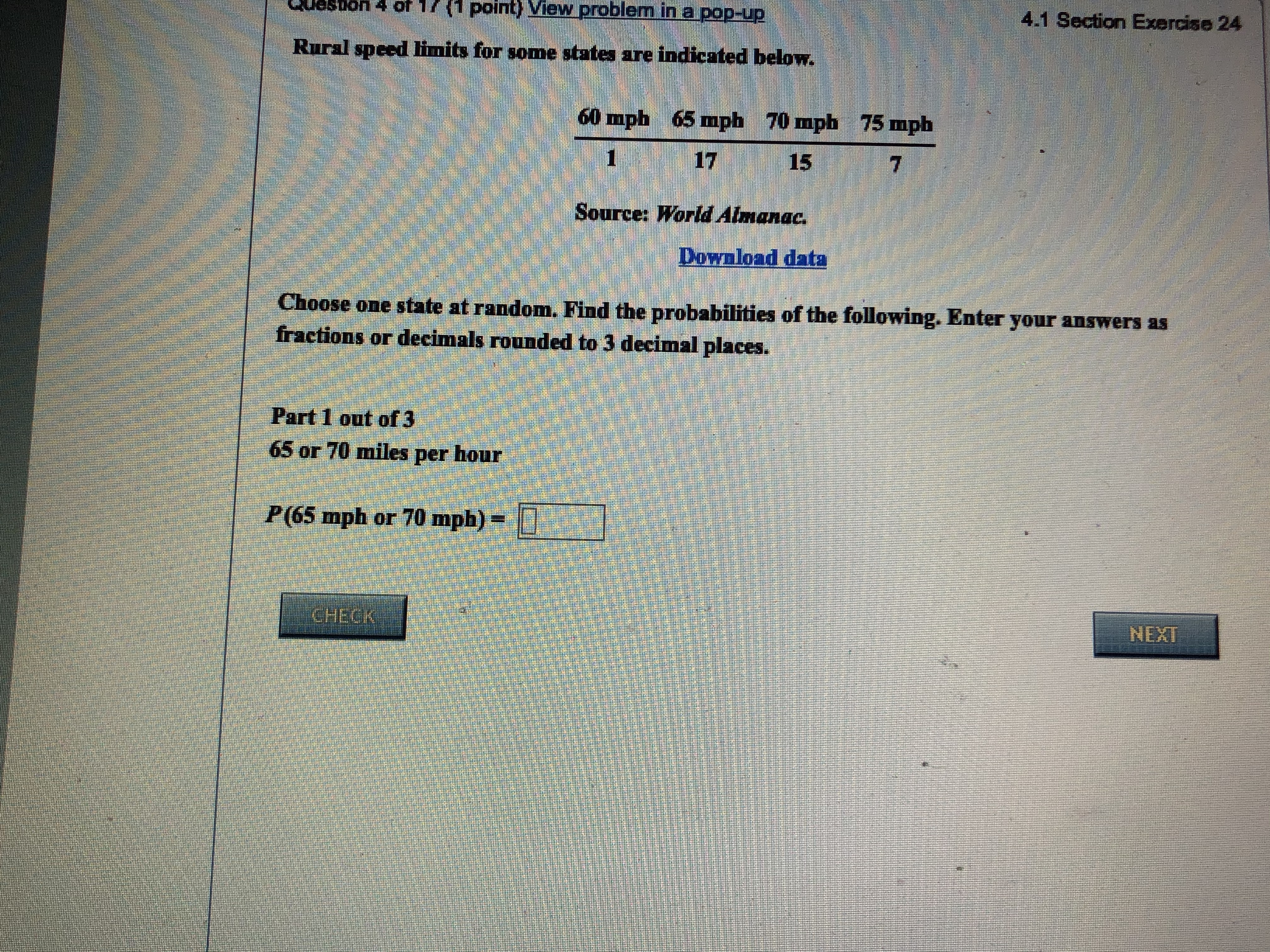17 (1 point) View problem in a pop-up4.1 Section Exercise 24OSDON 4 ORural speed limits for some states are indicated below.60 mph65 mph 70 mph75 mph17715Source: World AimanacDownload dataChoose one state at randonm. Find the probabilities of the following. Enter your answers asfractions or decimals rounded to 3 decimal places.Part 1 out of 365 or 70 miles per hourP(65 mph or 70 mph)CHECKNEXT

Questionhelp_outlineImage Transcriptionclose17 (1 point) View problem in a pop-up 4.1 Section Exercise 24 OSDON 4 O Rural speed limits for some states are indicated below. 60 mph 65 mph 70 mph 75 mph 17 7 15 Source: World Aimanac Download data Choose one state at randonm. Find the probabilities of the following. Enter your answers as fractions or decimals rounded to 3 decimal places. Part 1 out of 3 65 or 70 miles per hour P(65 mph or 70 mph) CHECK NEXT fullscreen
Step 1

Introduction:

Probability of an event is the number of cases favorable to the event, divided by the total number of possible cases. For an event A, P (A) = n (A) / n, where n (A) is the number of cases favorable to A, and n is the total number of possible cases.

Here, total number of possible cases, n = 1 + 17 + 15 + 7 = 40.

Number of state...

Want to see the full answer?

See Solution

Want to see this answer and more?

Our solutions are written by experts, many with advanced degrees, and available 24/7

See Solution
Tagged in

Statistics